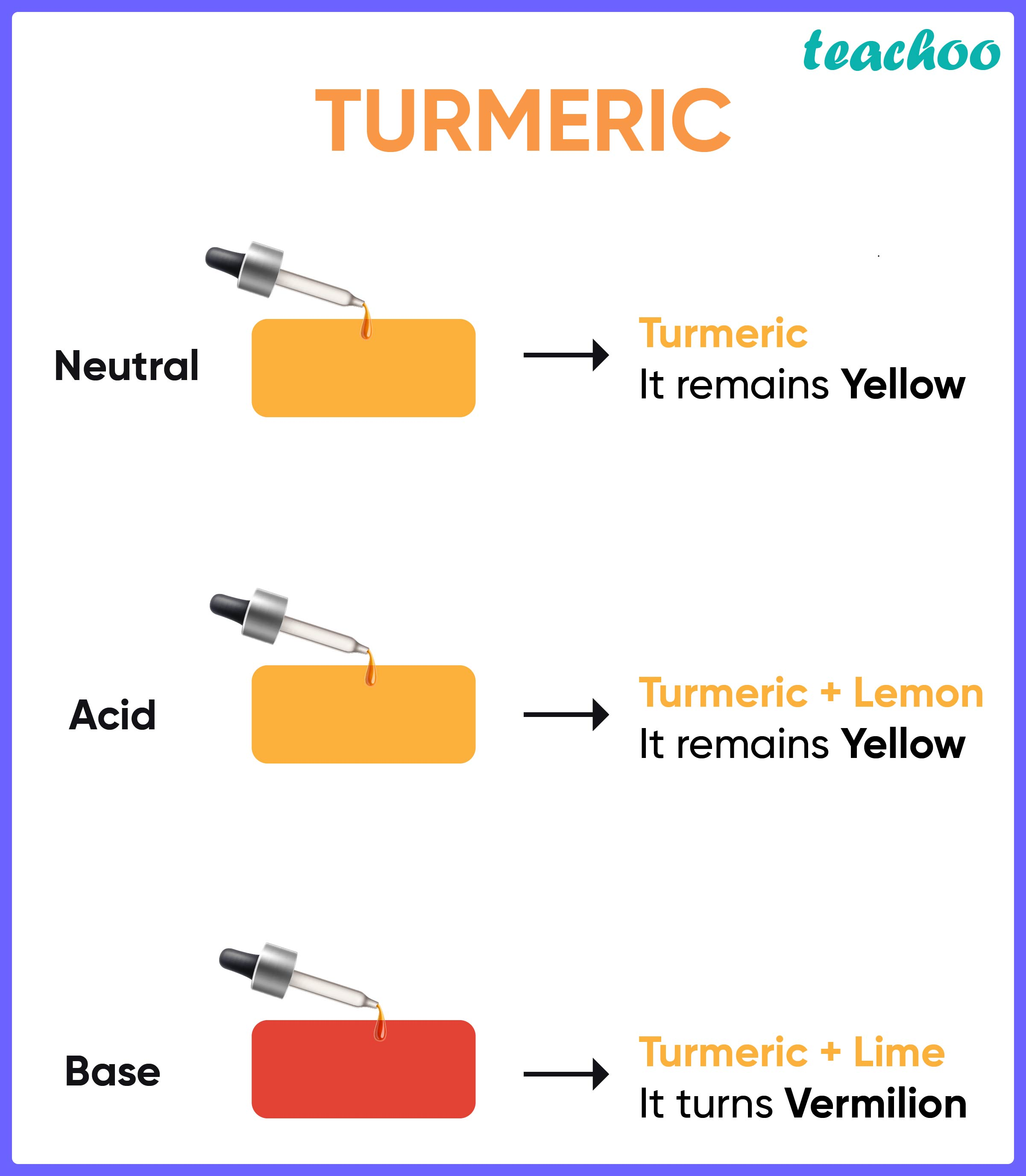NCERT Questions

Class 7
Chapter 5 Class 7 - Acid, Bases and Salt

## Three liquids are given to you. One is hydrochloric acid, another is sodium hydroxide and the third is a sugar solution. How will you identify them? You have only turmeric indicator

A turmeric indicator is a natural indicator which works as follows:

1. It does not change its colour when acidic or neutral substances are tested.
2. Its colour changes to bright red when basic substances are tested.To test the substances,

We will put a drop each of hydrochloric acid, sodium hydroxide, and sugar solution on the turmeric indicator.

• The liquid which changes the colour of turmeric indicator to red is basic in nature, that is, sodium hydroxide
• Hence sodium hydroxide is identified.

Now, we'll mix a drop of sodium hydroxide with a drop of the other two solutions and then perform the test again.

1.

• If the resultant turns the turmeric red , it is a basic solution.
• We know that, (Basic solution + Neutral solution) = Basic solution
• (Sodium hydroxide + sugar) = Basic solution
• Hence this mixture contains the sugar solution.

2.

• If the resultant does not change the colour of the turmeric paper at all,
• It is a neutral solution formed due to the neutralisation reaction between Sodium Hydroxide and Hydrochloric Acid
•   (Sodium hydroxide + hydrochloric acid) = Neutral
• Hence this mixture would contain hydrochloric acid.

Learn in your speed, with individual attention - Teachoo Maths 1-on-1 Class